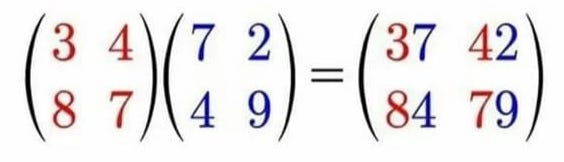# Finding Matrices that you can multiply wrong, rightMy codegolf.stackexchange post

Github Repository with experiments

I went on an adventure finding NxN matrices $A$ and $B$ where $AB = 10A + B$.

## Symbolic work

First of all, for a given candidate A, B is fixed:

$AB = 10A + B\\ A = 10AB^{-1} + I\\ I = 10B^{-1} + A^{-1}\\ B = 10(I - A^{-1})^{-1}\\ B = 10(I + (A - I)^{-1})$

One approach is to take the eigendecomposition of A.

From before,

$A = Q \Lambda_A Q^{-1}\\ B = 10(I - A^{-1})^{-1}\\ B = 10(Q I Q^{-1} - Q \Lambda_A^{-1} Q^{-1})^{-1}\\ B = Q(\frac{10}{(I - \Lambda_A^{-1})})Q^{-1}\\$

This shows that A, B share eigenvectors Q, and their eigenvalues are assosciated by

$\Lambda_A \Lambda_B = 10 \Lambda_A + \Lambda_B\\$

Interestingly, this proves that AB = BA, ie our matrices commute!

We can calculate the determinant of B as $10^N \frac{1}{\Pi_i (1 - \frac{1}{\lambda_{A,i}})}$ This is very suggestive, but doesn't immediately yield a way to pick a matrix A such that B is small positive integers.

Because A and B share eigenvectors, B can be written as a linear combination of $(I, A, A^2 ... ~ A^{N-1})$. For example,

$A = \left(\begin{array}{rrr}1 & 2 & 4 \\1 & 1 & 3 \\1 & 1 & 1\end{array}\right), B = \left(\begin{array}{rrr}7 & 4 & 6 \\3 & 6 & 4 \\1 & 2 & 8\end{array}\right)$ $\Lambda_A = \left(\begin{array}{rrr}-\sqrt{6} + 2 & 0 & 0 \\0 & \sqrt{6} + 2 & 0 \\0 & 0 & -1\end{array}\right), \Lambda_B = \left(\begin{array}{rrr}-\sqrt{6} + 2 & 0 & 0 \\0 & \sqrt{6} + 2 & 0 \\0 & 0 & -1\end{array}\right)$

We observe that $\Lambda_B = \Lambda_A^2 - 2 \Lambda_A + 2I$, so $B = A^2 - 2A + 2$. In this case B is forced to be an integer matrix because it is possible to write B as an integer polynomial of A. If we can find large matrices where $10(I + (\Lambda_A - I)^{-1})$ is an integer polynomial of $\Lambda_A$, then that might provide an efficient solution.

Finally, if we write $A$ as $E + I$, then we can see some simplifications. $B = 10 * (E^{-1} + I)$, so B is an integer matrix as long as the determinant of E divides 10. With this E representation, our problem can become to find an integer matrix E such that

$E + I \geq 0\\ E^{-1} + I \geq 0\\ |E| = \pm 10$

(Occasionally this will fail if an entry of $E^{-1} + I > 9$ )

This represents an improvement, because once we find a [0-9] matrix E with determinant $10$ (such as by random search), then if there are only a small number of negative entries in $E^{-1}$ we can run determinant-preserving transformations on E such as permuting rows to move those negative entries onto the diagonal, where they are made positive by the $+I$

In addition, there is a name and wikipedia page for matrices like $E^{-1}$ where the off diagonal entries are all positive: A Metzler Matrix. Further literature search in that direction might bring up an efficient way to generate positive integer matrices with a determinant $\pm 10$ whose inverses are Metzler Matrices.

subdavis.com forrestli.com